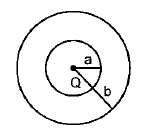# The region between two concentric spheres of radii 'a' and 'b', respectively(see figure), has volume charge density ρ=Ar , where A is a constant and r is the distance from the centre. At the centre of the spheres is a point charge Q. The value of A such that the electric field in the region between the spheres will be constant, is :Solution in Bengali

A

2Qπa2

B

Q2πa2

C

Q2π(b2a2)

D

2Qπ(b2a2)

Updated on:21/07/2023
Text Solution
Verified by Experts